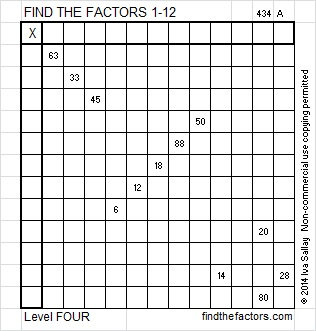# 216 and Level 4

Contents

### Today’s Puzzle:

It’s been a busy summer for me and lots of other people, yet Alan Parr found the time this summer to write a great post about the Find the Factors puzzles.  You can read it at established1962 find-the-factors.Excel file of puzzles and previous week’s factor solutions: 12 Factors 2014-08-25

### Steps That Use Logic to Solve That Puzzle:### Factors of 216:

• 216 is a composite number.
• 216 = 6 x 6 x 6, so it is a perfect cube, 6³.
• Prime factorization: 216 = 2 x 2 x 2 x 3 x 3 x 3, which can be written 216 = 2³ x 3³.
• The exponents in the prime factorization are 3 and 3. Adding one to each and multiplying we get (3 + 1) x (3 + 1) = 4 x 4 = 16. Therefore 216 has 16 factors.
• Factors of 216: 1, 2, 3, 4, 6, 8, 9, 12, 18, 24, 27, 36, 54, 72, 108, 216
• Factor pairs: 216 = 1 x 216, 2 x 108, 3 x 72, 4 x 54, 6 x 36, 8 x 27, 9 x 24, 12 x 18
• Taking the factor pair with the largest square number factor, we get √216 = (√6)(√36) = 6√6 ≈ 14.697### Sum-Difference Puzzle:

54 has four factor pairs. One of those factor pairs adds up to 15, and another one subtracts to 15. Those factor pairs will help you solve the first puzzle below.

216 has eight factor pairs. One of them adds up to 30 and a different one subtracts to 30. If you can identify those factor pairs, then you can solve the second puzzle.The second puzzle is really just the first puzzle in disguise. Why did I say that?

This site uses Akismet to reduce spam. Learn how your comment data is processed.